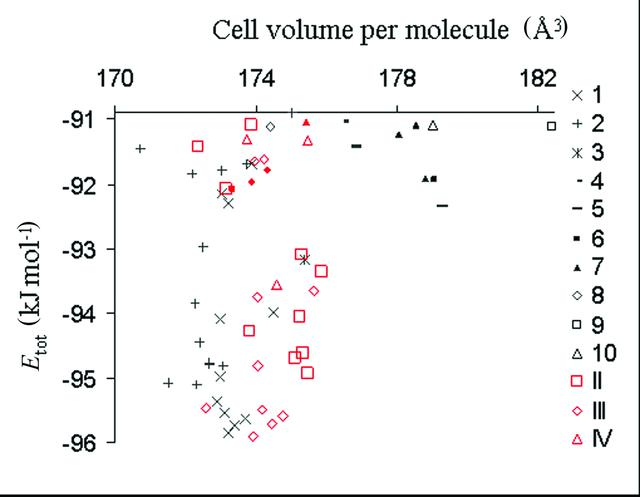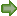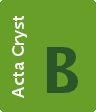disable zoom     view article Figure 6 Crystal structures within 5 kJ mol−1 of the global minimum in terms of Etot. Each symbol represents a different structure type as defined in the key. Black points are calculated hypothetical structure types (1–10) and red points are calculated structures types that have been observed experimentally (red squares, diamonds and triangles represent type II, III and IV structures, respectively). Results of expmincon calculations (solid red points, see Table 2) do not correspond exactly to structures found in the searches (hollow red points) because θ1 and θ2 were constrained to the exact experimental values in expmincon calculations. Form I calculated structures are outside the energy range in this plot.STRUCTURAL SCIENCECRYSTAL ENGINEERINGMATERIALS
ISSN: 2052-5206
Volume 61| Part 5| September 2005| Pages 558-568